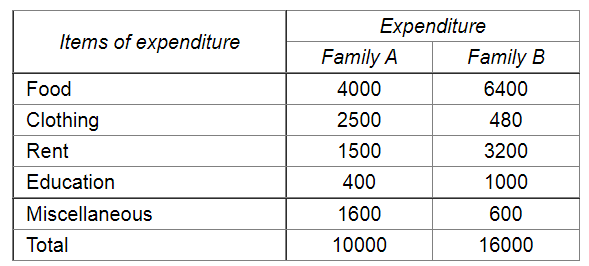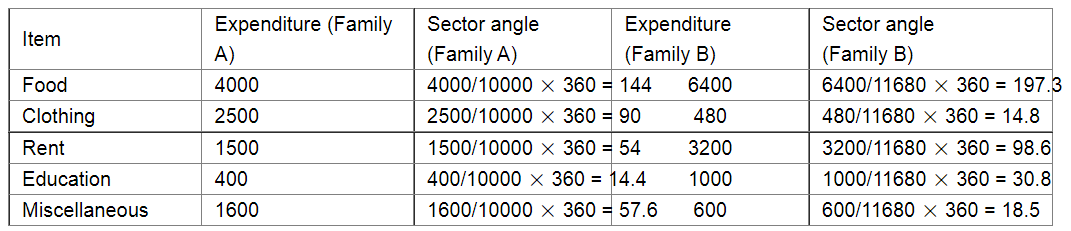# Represent the following data by a pie-diagram:

Question:

Represent the following data by a pie-diagram:Solution:

We know:

Central angle of a component $=\left(\right.$ component value $/$ sum of component values $\left.\times 360^{\circ}\right)$

Here the total expenditure of family A = 10000 and family B = 11680

Thus the central angle for each component can be calculated as follows:Total expenditure of family A: 10000
Total expenditure of family B: 11680  (not 16000)

Now, the pie chat representing the given data can be constructed by following the steps below:

Step 1 : Draw circle of an appropriate radius.

Step 2 : Draw a vertical radius of the circle drawn in step 1.

Step 3 : Choose the largest central angle. Here the largest central angle is 144o. Draw a sector with the central angle 144o in such a way that one of its radii coincides with the radius drawn in step 2 and another radius is in its counter clockwise direction.

Step 4 : Construct other sectors representing the other items in the clockwise sense in descending order of magnitudes of their central angles.

Step 5 : Shade the sectors with different colours and label them, as shown as in figure below.​​

Family AFamily B